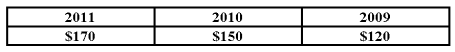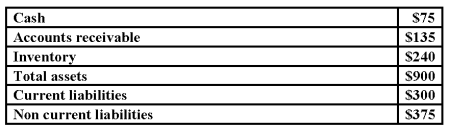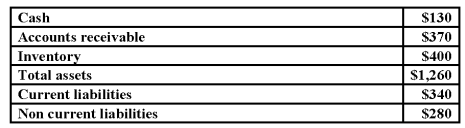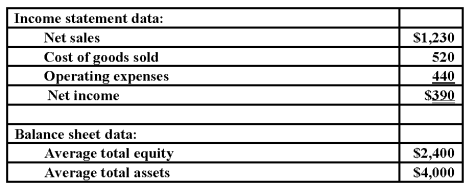/
/
/
91. During the years 2009 through 2011, Powers, Inc., reported the
Not my Question
Flag Content

# Question : 91. During the years 2009 through 2011, Powers, Inc., reported the : 1267474

91. During the years 2009 through 2011, Powers, Inc., reported the following amounts of net income (dollars in thousands):Relative to the prior year, the percentage change in net income:
A. Was the same in 2010 and 2011.
B. Was larger in 2011 than in 2010.
C. Was smaller in 2011 than in 2010.
D. Cannot be determined without knowing how many shares of stock were outstanding.

Shown below are selected data from the balance sheet of Compros, a small electronics store (dollar amounts are in thousands):92. Refer to the above data. What is the quick ratio?
A. 1.5 to 1.
B. .7 to 1.
C. .45 to 1.
D. Some other amount.

93. Refer to the above data. What is the current ratio?
A. 5.0 to 1.
B. 1.5 to 1.
C. .7 to 1.
D. Some other amount.

94. Refer to the above data. What is working capital amounts to:
A. \$225,000.
B. \$300,000.
C. \$150,000.
D. Some other amount.

95. Refer to the above data. What is Compro's debt ratio?
A. 75%.
B. 25%.
C. 60%.
D. Some other amount.

Shown below are selected data from the balance sheet of Bill's Auto Parts, a retail store (dollar amounts are in thousands):96. Refer to the above data. What is the quick ratio?
A. 5%.
B. 1.5 to 1.
C. 20%.
D. Some other amount.

97. Refer to the above data. What is the current ratio?
A. 1.2 to 1.
B. Less than 2 to 1, but not 1.2 to 1.
C. 2.6 to 1.
D. More than 2 to 1, but not 2.6 to 1.

98. Refer to the above data. Working capital amounts to:
A. \$560,000
B. \$530,000
C. \$270,000
D. Some other amount.

99. Refer to the above data. Bill's debt ratio is:
A. 22%.
B. 27%.
C. 57%.
D. Some other amount.

Shown below are selected data from the financial statements of Supreme Co. Dollar amounts are in millions (except for the per share data).Per share data (these amounts stated in actual dollars, not millions):
Supreme reported earnings per share for the year of \$4 and paid cash dividends of \$1 per share. At year end, the Wall Street Journal listed Supreme's capital stock as trading at \$88 per share.

100. Refer to the above data. Supreme's gross profit rate was:
A. 42.9%.
B. 57.7%.
C. 20.0%.
D. Some other amount.

## Solution 5 (1 Ratings )

Solved
Accounting 10 Months Ago 95 Views